# Recursive Sequence: Formula & Overview

Lesson Transcript
Instructor: Yuanxin (Amy) Yang Alcocer

Amy has a master's degree in secondary education and has taught math at a public charter high school.

One of the most famous recursive sequences is the Fibonacci sequence. In this lesson, learn what makes the Fibonacci sequence a recursive sequence, and discover how you can recognize and create your own. Updated: 05/06/2021

## Recursive Sequence: Definition

A recursive sequence is a sequence where the next terms use the previous terms. Let's take a look at the famous Fibonacci sequence to see what that means.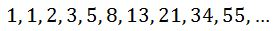This famous sequence is recursive because each term after the second term is the sum of the previous two terms. Our first two terms are 1 and 1. The third term is the previous two terms added together, or 1 + 1 = 2. The next term is the addition of the two prior terms, or 1 + 2 = 3. And this pattern continues indefinitely.

Let's see what this looks like in formula form.

An error occurred trying to load this video.

Try refreshing the page, or contact customer support.

Coming up next: Using a Graph to Define Limits

### You're on a roll. Keep up the good work!

Replay
Your next lesson will play in 10 seconds
• 0:04 Recursive Sequence Definition
• 0:49 Recursive Sequence Formulas
• 1:40 Using the Formulas
• 3:39 Finding Patterns
• 5:08 Lesson Summary
Save Save

Want to watch this again later?

Log in or sign up to add this lesson to a Custom Course.

Timeline
Autoplay
Autoplay
Speed Speed

## Recursive Sequence: Formulas

The Fibonacci sequence will look like this in formula form: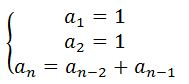Each term is labeled as the lowercase letter a with a subscript denoting which number in the sequence the term is. Lower case a1 is the first number in the sequence. Lower case a2 is the second number in the sequence and so on.

Recursive sequences do not have one common formula. You will have one formula for each unique type of recursive sequence.

There is one thing that recursive formulas will have in common, though: that is that each formula will have a sub n equal to a formula involving a sub some previous term. It doesn't have to be the n - 1 term or the previous term. It could be the third previous term. It could be any previous term. But the formula has to have a previous term in it to make it recursive.

## Using the Formulas

Using formulas requires you to know the starting terms and to plug these terms into the formula to calculate the next terms. Let's see how this works out with the following recursive formula appearing here: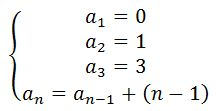This formula is a bit tricky because we have n - 1 in our formula. What does this mean? We know what the a(n-1) means. It means the previous term.

So, what could n - 1 possibly mean? Hmmm. What would happen if we simply plugged in my value for n and evaluated it like that? Yes! That is exactly what the n - 1 means. It wants you to take your current position in the sequence and subtract 1 from it.

If you are figuring out the fourth term in the sequence, it is asking you to take 4 and subtract 1 from it to get 3. This means we are adding 3 to the previous term, the third term. Our fourth term is then 6. Looking at our first three terms, we can also ask ourselves if these numbers follow the same pattern. The second number in the sequence is a 1. Our position at this point is 2, and if we subtract 1 from it we get 1. If we add this to our previous term, we get 1. Does this match our second term? Yes, it does, actually. Looking at the third term and asking the same question, we see that it also works with our pattern.

Here is a simpler formula to try out: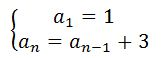This formula is telling us to add 3 to our previous term. Our first term is 1, so our next term will be 1 + 3 = 4. The third term will be 4 + 3 = 7. Our sequence will look like this: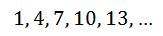To unlock this lesson you must be a Study.com Member.
Create your account

### Register to view this lesson

Are you a student or a teacher?

### Unlock Your Education

#### See for yourself why 30 million people use Study.com

##### Become a Study.com member and start learning now.
Back
What teachers are saying about Study.com
Create an account to start this course today
Used by over 30 million students worldwide